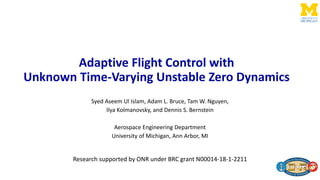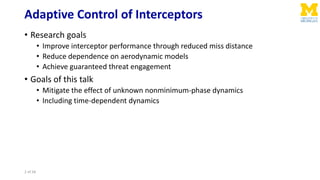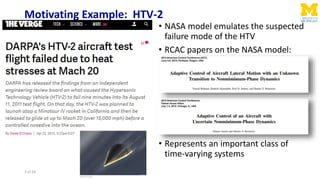Successfully reported this slideshow.

# Adaptive Flight-Control with Unknown Time-Varying Unstable Zero Dynamics×

1 of 28
1 of 28

# Adaptive Flight-Control with Unknown Time-Varying Unstable Zero Dynamics

SciTech 2020

SciTech 2020

## More Related Content

### Adaptive Flight-Control with Unknown Time-Varying Unstable Zero Dynamics

1. 1. Adaptive Flight Control with Unknown Time-Varying Unstable Zero Dynamics Syed Aseem Ul Islam, Adam L. Bruce, Tam W. Nguyen, Ilya Kolmanovsky, and Dennis S. Bernstein Aerospace Engineering Department University of Michigan, Ann Arbor, MI Research supported by ONR under BRC grant N00014-18-1-2211
2. 2. Adaptive Control of Interceptors • Research goals • Improve interceptor performance through reduced miss distance • Reduce dependence on aerodynamic models • Achieve guaranteed threat engagement • Goals of this talk • Mitigate the effect of unknown nonminimum-phase dynamics • Including time-dependent dynamics 2 of 28
3. 3. Motivating Example: HTV-2 • NASA model emulates the suspected failure mode of the HTV • RCAC papers on the NASA model: • Represents an important class of time-varying systems 3 of 28
4. 4. Time-Varying NMP Zeros • Unknown transition from minimum-phase to NMP dynamics • Emulates HTV failure mode (asymmetric ablation) Continuous time Discrete time Plant at 𝑡1 is unknown It is MP Time of transition is unknown Plant at 𝑡2 is unknown It is NMP Transfer function from aileron to roll angle 4 of 28
5. 5. Approach • Use retrospective cost adaptive control (RCAC) to follow roll angle commands • RCAC is effective for LTI NMP plants with known NMP zeros • RCAC may cancel unknown NMP zeros • RCAC is effective on time-varying plants if the time dependence is not too fast • Solution: Data-driven RCAC (DDRCAC) • Perform concurrent online identification • Extract closed-loop target model for use by RCAC • To be explained • Investigate performance on time-varying plants 5 of 28
6. 6. Adaptive Standard Problem for RCAC Controller is linear with time-varying coefficients that are adapted Performance variable Command or disturbance With full-state feedback (𝑦 = 𝑥), 𝐺𝑧𝑢 can still be NMP All examples in this talk are output feedback (only the performance variable z is measured and y = z) 6 of 28
7. 7. RCAC Input-Output Controller Structure • Discrete-time MIMO Input-Output Controller 𝑢 𝑘 = 𝑖=1 ℓc 𝑃𝑖,𝑘 𝑢 𝑘−𝑖 + 𝑖=1 ℓc 𝑄𝑖,𝑘 𝑦 𝑘−𝑖 • Specialization to SISO controller 𝑢 𝑘 = 𝜙 𝑘 𝜃 𝑘 = 𝑢 𝑘 ⋯ 𝑢 𝑘−ℓc 𝑦 𝑘−1 ⋯ 𝑦 𝑘−ℓc 𝑃1,𝑘 ⋮ 𝑃ℓc,𝑘 𝑄1,𝑘 ⋮ 𝑄ℓc,𝑘 𝐺c,𝑘 𝐪 = 𝑄1,𝑘 𝐪ℓc−1 + ⋯ + 𝑄ℓc,𝑘 𝐪ℓc − 𝑃1,𝑘 𝐪ℓc−1 − ⋯ − 𝑃ℓc,𝑘 = 𝑁c,𝑘(𝐪) 𝐷c,𝑘(𝐪) Regressor Controller Coefficients Time-Domain Representation Controller coefficients are optimized at each step 7 of 28
8. 8. • Optimization drives the retrospective performance variable to zero 𝑧 𝑘 𝜃 ≜ 𝑧 𝑘 − 𝐺f 𝐪 [𝑢 𝑘 − 𝜙 𝑘 𝜃] 𝑧 𝑘 = 𝐺 𝑘 𝐪 𝑣0,𝑘( 𝜃) 𝑧 𝑘 ≈ 𝐺f 𝐪 𝑣0,𝑘( 𝜃) How Does RCAC Work? Minimization of 𝑧 𝑘 “drives” 𝐺 𝑘 to 𝐺f Target model 𝜙 𝑘 𝜃 Controller Denominator Controller Numerator 𝐺c,𝑘 = 𝑁c,𝑘 𝐷c,𝑘 Intercalated injection 𝑢 𝑘 𝑧 𝑘 𝑣0,𝑘( 𝜃) 𝑤 𝑘 𝑦 𝑘 𝐺 𝑘 𝐪 = 𝑁𝑧𝑢(𝐪)𝐪 𝑛c 𝐷c,𝑘 𝐪 𝐷 𝐪 − 𝑁 𝑦𝑢 𝐪 𝐺c,𝑘 𝐪 Actual transfer function from 𝒗 𝟎,𝒌 to 𝒛 𝒌 𝑮 𝐟 must: • Include all NMP zeros of 𝑮 𝒛𝒖 • Match the relative degree of 𝑮 𝒛𝒖 • Match the sign of 𝑮 𝒛𝒖 8 of 28
9. 9. Recursive Least Squares (RLS) 𝑧 𝑘 𝜃 ≜ 𝑧 𝑘 − 𝐺f(𝐪) 𝑢 𝑘 − 𝜙 𝑘 𝜃 𝐽 𝑘 𝜃 ≜ 𝑖=1 𝑘 𝜆 𝑘−𝑖 𝑧𝑖 T 𝜃 𝑅 𝑧 𝑧𝑖 𝜃 + 𝜆 𝑘 𝜃 − 𝜃0 T 𝑃0 −1 𝜃 − 𝜃0 𝜃 𝑘+1 ≜ argmin 𝜃 𝐽 𝑘 𝜃 Forgetting Factor This is not computed 𝑃𝑘+1 = 1 𝜆 𝑃𝑘 − 1 𝜆 𝑃𝑘Φ 𝑘 T 𝑁T 𝜆𝑅 𝑧 −1 + 𝑁Φ 𝑘 𝑃𝑘Φ 𝑘 T 𝑁T −1 𝑁Φ 𝑘 𝑃𝑘 𝜃 𝑘+1 = 𝜃 𝑘 − 𝑃𝑘Φ 𝑘 T 𝑁T 𝑅 𝑧 𝑁Φ 𝑘 𝜃 𝑘 + 𝑧 𝑘 − 𝑁 𝑈 𝑘 𝑙 𝑧 × 𝑙 𝑧 inverse𝑙 𝜃 × 𝑙 𝜃 matrix 𝑛f 𝑙 𝑢 × 𝑙 𝜃 data buffer 𝑛f 𝑙 𝑢 × 1 data buffer 𝑙 𝑧 × 𝑛f 𝑙 𝑢 filter matrix 𝑙 𝜃 × 1 controller coefficient vector RLS This is implemented 9 of 28
10. 10. RCAC with MP Plant • RCAC follows step command with rudimentary target model 𝐺f x 𝐺c,𝑘 x o 𝐺 𝑧 converges to 0 𝐺 𝐪 = (𝐪 − 0.3)(𝐪 − 0.7) (𝐪 − 1.1)(𝐪2 − 1.4𝐪 + 1.052) 𝐺f 𝐪 = 1 𝐪 10 of 28
11. 11. RCAC with Unmodeled NMP Zeros • RCAC cancels unmodeled or poorly modeled NMP zeros under sufficiently high authority x 𝐺c,𝑘 x o 𝐺 Unmodeled NMP zero cancelled by RCAC Distance from NMP zero to closest controller pole 𝑧 diverges 𝑢 diverges 11 of 28 𝐺 𝐪 = 𝐪 − 1.3 𝐪2 − 0.7𝐪 + 0.48) 𝐺f 𝐪 = 1 𝐪
12. 12. Motivation for Data-Driven RCAC • All NMP zeros of the plant (𝐺𝑧𝑢) must be modeled • But these are unknown and time-varying in the HTV application • The time of transition of the plant from MP to NMP is unknown • Ad-hoc fixes to update 𝐺f are not practical • DDRCAC: Identify rudimentary model online using RLS • Extract information needed for 𝐺f 𝑮 𝐟 must: • Include all NMP zeros of 𝑮 𝒛𝒖 • Match the relative degree of 𝑮 𝒛𝒖 • Match the sign of 𝑮 𝒛𝒖 12 of 28
13. 13. Data-Driven Retrospective Cost Adaptive Control • Fit an IO model to 𝑦 𝑘, 𝑢 𝑘 • Use RLS to minimize 𝑦 𝑘 + 𝑖 𝜂 𝐹𝑖,𝑘 𝑦 𝑘−𝑖 − 𝑖 𝜂 𝐺𝑖.𝑘 𝑢 𝑘−𝑖 • Yields estimates 𝐹𝑖,𝑘, 𝐺𝑖,𝑘 • Use 𝐺𝑖, discard 𝐹𝑖 • 𝐺f,𝑘 𝐪 = 𝑖 𝜂 1 𝐪 𝑖 𝐺𝑖,𝑘 • 𝐺f,𝑘 is FIR • 𝑧 𝑘 ≜ 𝑧 𝑘 − 𝐺f,𝑘(𝜙 𝑘 𝜃 − 𝑢 𝑘) • Minimize 𝑖=0 𝑘 𝑧𝑖 T 𝑧𝑖 + 𝜙 𝑘 𝜃 − 𝑢 𝑘 T 𝑅 𝑢(𝜙 𝑘 𝜃 − 𝑢 𝑘) • Yields updated controller coefficients 𝜃 𝑘+1 System Identification (RLSID) RCAC Captures leading sign, NMP zeros, relative degree of 𝑮 𝒛𝒖 13 of 28
14. 14. RLS with Variable-Rate Forgetting Let 𝜆 𝑘 ∈ (0,1], define 𝜌 𝑘 ≜ Π𝑗=0 𝑘 𝜆𝑗 and 𝐽 𝑘(Θ) ≜ 𝑖=0 𝑘 𝜌 𝑘 𝜌𝑖 𝑌𝑖 − Φ𝑖Θ T 𝑌𝑖 − Φ𝑖Θ + 𝜌 𝑘 Θ − Θ0 T 𝑃0 −1 Θ − Θ0 The minimizer Θ 𝑘+1 is given by 𝑃𝑘+1 = 1 𝜆 𝑘 𝑃𝑘 − 1 𝜆 𝑘 𝑃𝑘Φ 𝑘 T 𝜆 𝑘 𝐼𝑙 𝑌 + Φ 𝑘 𝑃𝑘Φ 𝑘 T −1 Φ 𝑘 𝑃𝑘 Θ 𝑘+1 = Θ 𝑘 + 𝑃𝑘+1Φ 𝑘 T (𝑌𝑘 − Φ 𝑘Θ 𝑘) Data-dependent VRF: 𝜆 𝑘 = 1 1 + 𝛾𝑓(𝑧 𝑘) where 𝑓(𝑧 𝑘) is 𝑓 𝑧 𝑘 = RMS 𝑧 𝑘−𝜏1 , … , 𝑧 𝑘 RMS 𝑧 𝑘−𝜏2 , … , 𝑧 𝑘 − 1, ratio > 1 0, otherwise 𝜆 𝑘 = 1 if 𝑧 𝑘 below noise floor Prevents forgetting due to sensor noise; promotes forgetting when the error is large Forgetting Factor Learning requires forgetting! 14 of 28
15. 15. Data-Driven Retrospective Cost Adaptive Control 𝑧 − 𝑟 𝑢 𝑦 𝑮 Online Identification Construct 𝑮 𝐟,𝒌 DDRCAC 𝑦 𝑢 Update 𝑮 𝐜 15 of 28
16. 16. DDRCAC Applied to a NMP Plant 2. Large transient leads to re-identification facilitated by VRF-ID RLSID RCAC Converged coefficients Zeros 4. Re-adaptation leads to command following3. Re-identification induces re-adaptation facilitated by VRF-AC Identifies NMP zero! 16 of 28 1. Initially poor model induces large transient x o Identified Model x o True System
17. 17. DDRCAC Hyperparameters 17 of 28
18. 18. Basic Servo Loop for Sampled-Data Control Sampled-data control: Continuous-time plant, controlled with a discrete-time controller 18 of 28
19. 19. SISO Example • Harmonic command following for SISO system with unknown transition from MP to NMP Zero move to RHP Lightly damped mode changes frequency 𝑤 𝑘 ∼ 𝑁 0,0.00012 , 𝑣 𝑘 ∼ 𝑁(0, 0.0012) 19 of 28
20. 20. SISO Example 𝑇𝑠 = 0.1, 𝜂 = 8, 𝑛c = 10, 𝑝0 = 1000, 𝑅 𝑢 = 0, 𝛾p = 𝛾c = 0.1, 𝛼 = 90, 𝜏1 = 40, 𝜏2 = 200 Unknown transition from MP to NMP happens 40 𝑠 < 𝑡 < 50 s (time of transition is also unknown) Unknown change in command frequency 20 of 28
21. 21. SISO Example Variable-rate forgettingVRF for RLSID VRF for RCAC Online-identification coefficients Adaptive controller coefficients High forgetting during and right after the transition21 of 28
22. 22. SIMO Example • Multi-step command following for SIMO system with unknown transition from stable MP to unstable NMP • Conflicting commands Transmission zeros move to RHP Plant becomes unstable 𝑤 𝑘 ∼ 𝑁 0,0.00012 , 𝑣 𝑘 ∼ 𝑁(0, 0.0012 )22 of 28
23. 23. SIMO Example 𝑇𝑠 = 0.1, 𝜂 = 8, 𝑛c = 10, 𝑝0 = 1000, 𝑅 𝑢 = 0, 𝛾p = 𝛾c = 0.1, 𝛼 = 100, 𝜏1 = 40, 𝜏2 = 200 Unknown transition from stable MP to unstable NMP happens 75 𝑠 < 𝑡 < 95 s (time of transition also unknown) Same hyperparameters as SISO example 23 of 28 Nonzero command is followed Zero command is impossible
24. 24. SIMO Example Variable-rate forgetting VRF for RLSID VRF for RCAC Online-identification coefficients Adaptive controller coefficients High forgetting during and right after transition24 of 28
25. 25. Hypersonic Aircraft: Lateral Dynamics Lightly damped mode changes frequency Complex MP zeros transition to two real zeros, one NMP Actuator Rate Saturation: 300 deg/s 𝑤 𝑘 ∼ 𝑁 0,0.00012 , 𝑣 𝑘 ∼ 𝑁(0, 0.0012) 25 of 28 𝑥 ≜ 𝛽 𝑝 𝑟 𝜙 ,
26. 26. Hypersonic Aircraft: Lateral Dynamics 𝑇𝑠 = 0.5, 𝜂 = 8, 𝑛c = 10, 𝑝0 = 1000, 𝑅 𝑢 = 0, 𝛾p = 𝛾c = 0.1, 𝛼 = 30∘, 𝜏1 = 40, 𝜏2 = 200 Unknown transition from MP to NMP happens 90 𝑠 < 𝑡 < 100 s (time of transition also unknown) All hyperparameters same as SISO and SIMO examples. Only sampling time and saturation level different. Unknown change in command frequency 26 of 28
27. 27. Hypersonic Aircraft: Lateral Dynamics Variable-rate forgetting VRF for RLSID VRF for RCAC Online-identification coefficients Adaptive controller coefficients High forgetting during and right after transition27 of 28
28. 28. Conclusions and Future Work • DDRCAC was used for linear time-varying plants that transition from MP to NMP • Step and harmonic commands • Unknown transition was estimated online using concurrent system identification • Performance was demonstrated in the presence of stochastic disturbances and sensor noise • The method was demonstrated for a NMP SIMO system • Applied to the lateral dynamics of a hypersonic aircraft • Ongoing work: • DDRCAC for plants with unknown nonlinear unstable zero dynamics. • DDRCAC for interceptor autopilot for guaranteed threat engagement 28 of 28

### Editor's Notes

• Say interceptor and threat as much as possible
• 2014 Paper: Shows RCAC doesn’t cause instability but no CF after transition OR NMP zero assumed known
2015 Paper: Exact locations of any NMP zeros assumed known OR transition time is known with an estimate of location of NMP zero
• Pls check and fix yellow box
• Point out NMP zeros of Gzu are a problem only, recall FSB
• Point out forgetting lambda
• Fix yellow box
• Results on next slide?